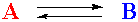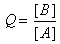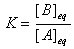# Equilibrium: Q and K

Consider a simple chemical system including just two compounds, A and B:This just says that if you mix A and B, A will react to become B, and B will react to become A. This equation also states that one molecule of A reacts to give one molecule of B. This will be an important part of the concept. A is just turning into B, and vice versa. No other substances are involved (presumably). Associated with this system are two quantities, Q, the reaction quotient, and K, the equilibrium constant.andIt is important to understand the distinction between Q and K.

• Q is a quantity that changes as a reaction system approaches equilibrium.

• K is the numerical value of Q at the "end" of the reaction, when equilibrium is reached.

 The goal of this web page is to help you understand this distinction between Q and K, and to let you explore the effect of changing the initial concentrations of A and B, the value of K, and the speed of the reaction on the final equilibrium state. Use the form shown on the right to adjust values and investigate the results. Several interesting questions to consider are given below. Each time you click the Check it out link, you will get a DIFFERENT set of initial conditions. So try it at least twice before you decide the answer to the question. Initial concentration of A mol/L Initial concentration of B mol/L Equilibrium constant, K Speed of the reaction 10 (fast) 9 8 7 6 5 4 3 2 1 (slow) React

1. Does Q change during the reaction, and if so, does it approach the value of K? Check it out

2. If K=1 and [B]>[A] initially, will [B] increase or decrease during the reaction? Check it out

3. If K=1 and [B]<[A] initially, will [B] increase or decrease during the reaction? Check it out

4. If K=5, [A]=0.2, and [B]=2.0, will [B] increase or decrease during the reaction? Check it out

5. If K=2, [A]=0.3, and [B]=0.6, will [B] increase or decrease during the reaction? Check it out